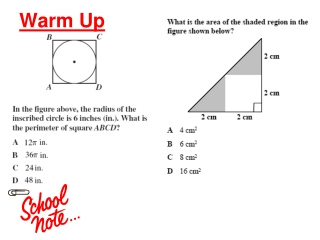Download PresentationWarm Up

# Warm Up

Download Presentation## Warm Up

- - - - - - - - - - - - - - - - - - - - - - - - - - - E N D - - - - - - - - - - - - - - - - - - - - - - - - - - -
##### Presentation Transcript

1. Warm Up

2. 11.6 Circumference and Arc Length

3. Finding circumference and arc length • The circumference of a circle is the distance around the circle. For all circles, the ratio of the circumference to the diameter is the same. This ratio is known as  or pi.

4. The circumference C of a circle is C = d or C = 2r, where d is the diameter of the circle and r is the radius of the circle. Theorem 11.6: Circumference of a Circle

5. And . . . • An arc length is a portion of the circumference of a circle. You can use the measure of an arc (in degrees) to find its length (in linear units).

6. Arc Length Corollary • In a circle, the ratio of the length of a given arc to the circumference is equal to the ratio of the measure of the arc to 360°. Arc length m = 2r 360° m Arc length = • 2r 360°

7. The length of a semicircle is half the circumference, and the length of a 90° arc is one quarter of the circumference. More . . . ½ • 2r r r ¼ • 2r

8. Ex. 2: Finding Arc Lengths • Find the length of each arc. a. b. c. 50° 100° 50°

9. • 2(5) a. Arc length of = 50° 360° Ex. 2: Finding Arc Lengths • Find the length of each arc. # of ° a. • 2r a. Arc length of = 360° 50°  4.36 centimeters

10. Ex. 2: Finding Arc Lengths • Find the length of each arc. # of ° b. • 2r b. Arc length of = 360° 50° 50° • 2(7) b. Arc length of = 360°  6.11 centimeters

11. Ex. 2: Finding Arc Lengths • Find the length of each arc. # of ° c. • 2r c. Arc length of = 360° 100° 100° • 2(7) c. Arc length of = 360°  12.22 centimeters

12. m Ex. 3: Using Arc Lengths • Find the indicated measure. a. circumference Arc length of = 2r 360° 3.82 = 60° 2r 360° 60° 3.82 1 = 2r 6 3.82(6) = 2r 22.92 = 2r C = 2r; so using substitution, C = 22.92 meters.

13. 10-7 Area of Circles and Sectors

14. Ex. 3: Using Arc Lengths • Find the indicated measure. b. m Arc length of m = 2r 360° 18 m = 360° • • 360° 2(7.64) 360° 135°  m

15. Theorem 10-11: Area of a Circle • The area of a circle is  times the square of the radius or A = r2.

16. Find the area of P. Solution: Use r = 8 in the area formula. A = r2 =  • 82 = 64  201.06 Ex. 1: Using the Area of a Circle • So, the area if 64, or about 201.06 square inches.

17. Find the diameter of Z. Solution: Area of circle Z is 96 cm2. A = r2 96=  r2 96= r2  30.56  r2 5.53  r Ex. 1: Using the Area of a Circle • The diameter of the circle is about 11.06 cm.

18. Theorem 10.12: Area of a Sector • The ratio of the area A of a sector of a circle to the area of the circle is equal to the ratio of the measure of the intercepted arc to 360°. m m A = or A = • r2 r2 360° 360°

19. Ex. 2: Finding the area of a sector • Find the area of the sector shown below. Sector CPD intercepts an arc whose measure is 80°. The radius is 4 ft. m A = • r2 360°

20. m A = • r2 360° 80° = • r2 360° Ex. 2 Solution Write the formula for area of a sector. Substitute known values. Use a calculator.  11.17 • So, the area of the sector is about 11.17 square feet.

21. Ex. 3: Finding the Area of a Sector • A and B are two points on a P with radius 9 inches and mAPB = 60°. Find the areas of the sectors formed by APB. 60°

22. Homework • Page 578 # 7 – 16, 20-21 & 43-45